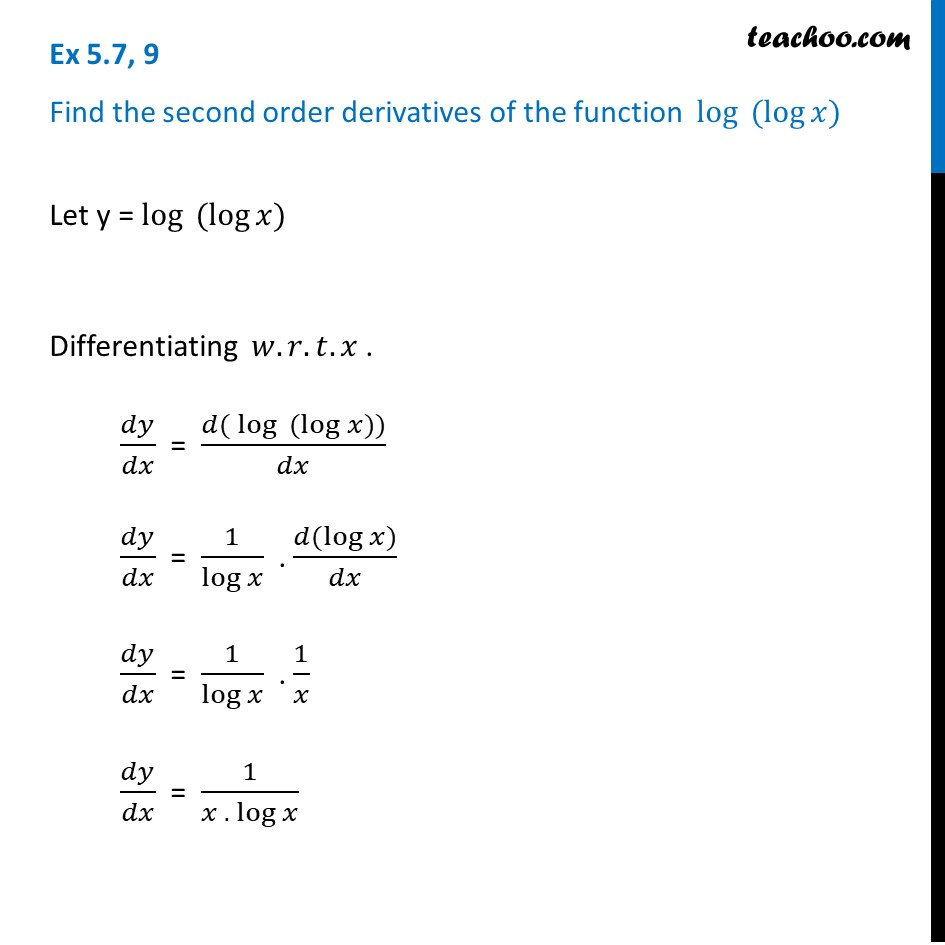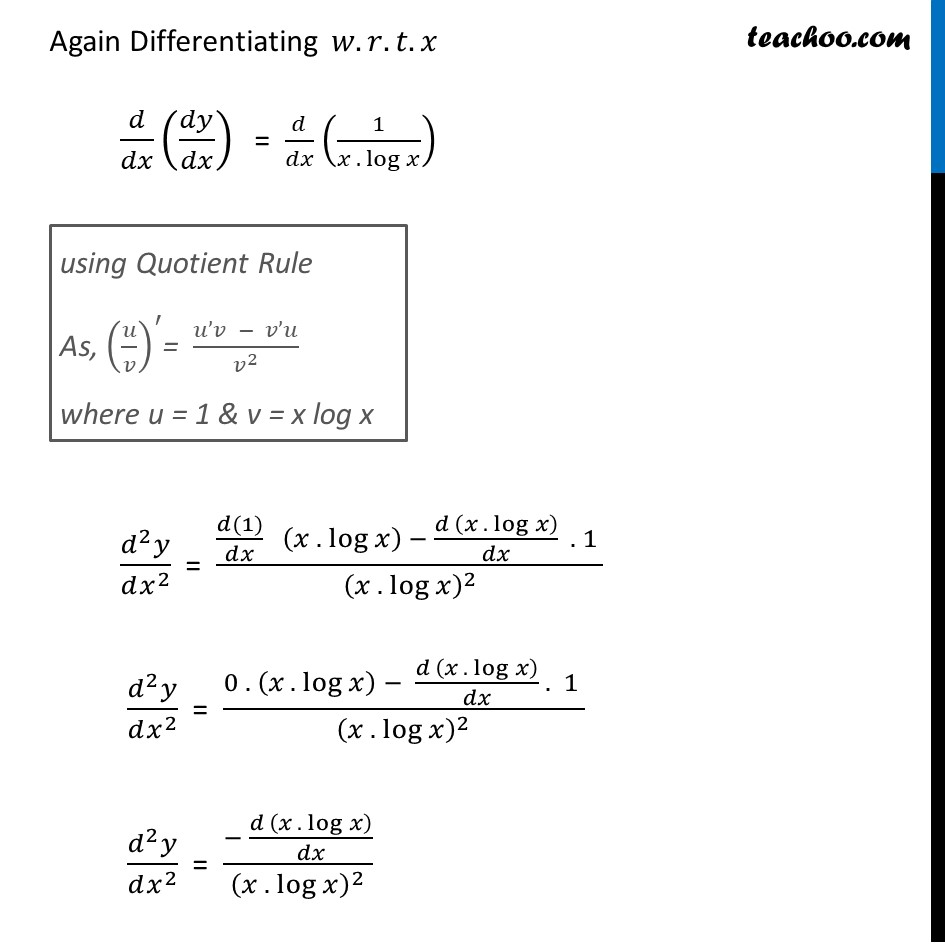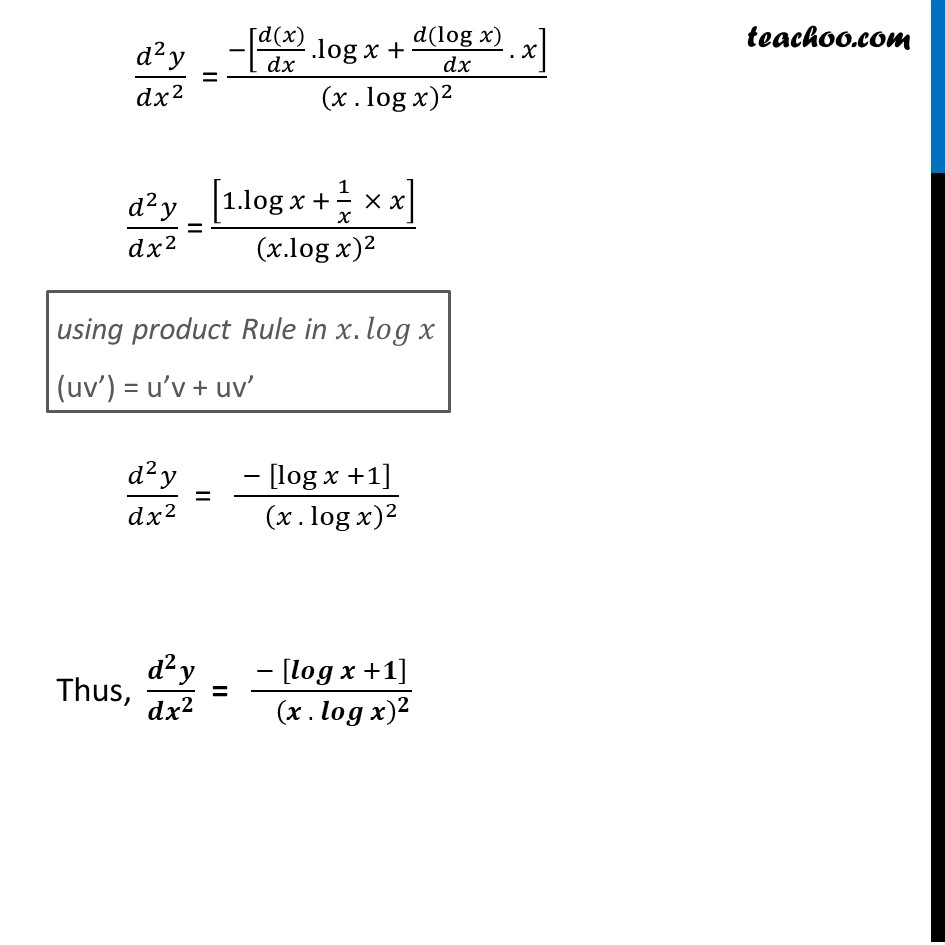Finding second order derivatives - Normal form

Chapter 5 Class 12 Continuity and Differentiability
Concept wiseIntroducing your new favourite teacher - Teachoo Black, at only ₹83 per month

### Transcript

Ex 5.7, 9 Find the second order derivatives of the function 〖 log〗⁡〖 (log⁡〖𝑥)〗 〗 Let y =〖 log〗⁡〖 (log⁡〖𝑥)〗 〗 Differentiating 𝑤.𝑟.𝑡.𝑥 . 𝑑𝑦/𝑑𝑥 = (𝑑(〖 log〗⁡〖 (log⁡〖𝑥)〗 〗))/𝑑𝑥 𝑑𝑦/𝑑𝑥 = 1/log⁡𝑥 . (𝑑(log⁡𝑥))/𝑑𝑥 𝑑𝑦/𝑑𝑥 = 1/log⁡𝑥 . 1/𝑥 𝑑𝑦/𝑑𝑥 = 1/〖𝑥 . log〗⁡𝑥 Again Differentiating 𝑤.𝑟.𝑡.𝑥 𝑑/𝑑𝑥 (𝑑𝑦/𝑑𝑥) = 𝑑/𝑑𝑥 (1/〖𝑥 . log〗⁡𝑥 ) (𝑑^2 𝑦)/(𝑑𝑥^2 ) = ((𝑑(1))/𝑑𝑥 (〖𝑥 . log〗⁡𝑥 ) − (𝑑 (〖𝑥 . log〗⁡𝑥 ))/𝑑𝑥 . 1 )/(〖𝑥 . log〗⁡𝑥 )^2 (𝑑^2 𝑦)/(𝑑𝑥^2 ) = (0 . (〖𝑥 . log〗⁡𝑥 ) − (𝑑 (〖𝑥 . log〗⁡𝑥 ))/𝑑𝑥 . 1 )/(〖𝑥 . log〗⁡𝑥 )^2 (𝑑^2 𝑦)/(𝑑𝑥^2 ) = (− (𝑑 (〖𝑥 . log〗⁡𝑥 ))/𝑑𝑥)/(〖𝑥 . log〗⁡𝑥 )^2 using Quotient Rule As, (𝑢/𝑣)^′= (𝑢’𝑣 − 𝑣’𝑢)/𝑣^2 where u = 1 & v = x log x (𝑑^2 𝑦)/(𝑑𝑥^2 ) = (−[(𝑑(𝑥))/𝑑𝑥 .log⁡𝑥 + (𝑑(log⁡〖𝑥)〗)/𝑑𝑥 . 𝑥])/(〖𝑥 . log〗⁡𝑥 )^2 (𝑑^2 𝑦)/(𝑑𝑥^2 ) = [1.log⁡〖𝑥 + 1/𝑥 × 𝑥〗 ]/( (𝑥.log⁡𝑥 )^2 ) (𝑑^2 𝑦)/(𝑑𝑥^2 ) = (− [log⁡𝑥 +1])/( (〖𝑥 . log〗⁡𝑥 )^2 ) Thus, (𝒅^𝟐 𝒚)/(𝒅𝒙^𝟐 ) = (− [𝒍𝒐𝒈⁡𝒙 +𝟏])/( (〖𝒙 . 𝒍𝒐𝒈〗⁡𝒙 )^𝟐 ) using product Rule in 〖𝑥. 𝑙𝑜𝑔〗⁡𝑥 (uv’) = u’v + uv’